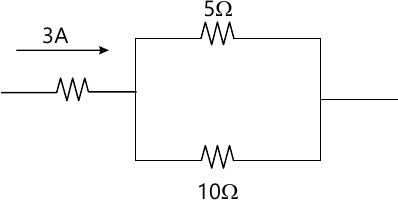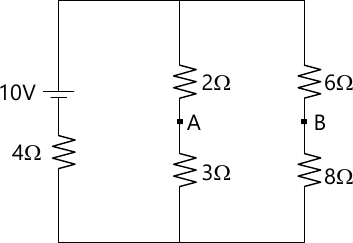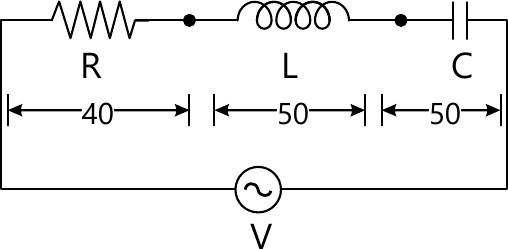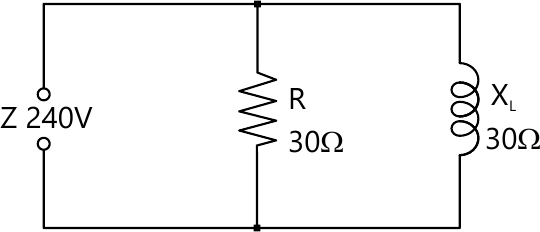MORE IN Basic Electrical Engg.
VTU First Year Engineering (P Cycle) (Semester 1)
Basic Electrical Engg.
June 2012
Total marks: --
Total time: --
INSTRUCTIONS
(1) Assume appropriate data and state your reasons
(2) Marks are given to the right of every question
(3) Draw neat diagrams wherever necessary

Choose the correct answers for the following
1(a)(i) The current in 5ohm resistor is
a) 2A b) 3A c) 1A d) 1.5A1 M
1(a)(ii) The total resistance of parallel circuit is
a) Less than the smallest resistance b) More than the smallest resistance c) More than the highest resistance d) None of these
1 M
1(a)(iii) Inductance opposes _________ in current in a circuit.
a) Only increase b) Only decrease c) Change d) None of these
1 M
1(a)(iv) If coefficient of coupling between two coils is increased, Mutual inductance between the coils _________
a) Is increased b) Is decreased c) Remains unchanged d) None of these
1 M
1(b) List out the advantages of parallel circuit over series circuit. List out the characteristics of parallel circuit.
6 M
1(c ) Deduce an expression for stored energy in a magnetic field.
4 M
1(d) Find current in the battery, the current in each branch and p.d. across AB in the network shown in figure.6 M

Choose the correct answers for the following
2(a)(i) A coil is rotating in the uniform field of a 10-pole generator. In one revolution of the coil, the number of cycles generated by voltage is __________
a) 10 b) 5 c) 2.5 d) 4
1 M
2(a)(ii) The average value of sine wave over one complete cycle is
a) Zero b) +1 c) -1 d) 1/2
1 M
2(a)(iii) The voltage of the applied source in the circuit of figure is
a) 50V b) 100V c) 40V d) 140V1 M
2(a)(iv) The power taken by the circuit shown is
a) 480W b) 1920W c) 1200W d) None of these1 M
2(b) With the help of circuit diagram and phasor diagram find the phase angle, impedance and power in case of R-L series circuit.
8 M
2(c) An alternating current of frequency 60Hz has a maximum value of 120A.
(i) Write down equation for the instantaneous value.
(ii) Reckoning time from the instant the current is zero and becoming positive, find the instantaneous value after 1/360 sec
(iii) Time taken to reach 96A for the first time.
4 M
2(d) A 60 ? resistor is connected in parallel with an inductive reactance of 80 ? to a 240V, 50Hz supply.
Calculate:
(i) The current through the resistor and inductance.
(ii) The supply current.
(iii) The circuit phase angle. Draw phasor diagram.
4 M

Choose the correct answers for the following
3(a)(i) Three inductive coils each having an impedance of 17.7 ? are connected in star. The circuit is fed from a 3-phase, 400V, and 50Hz supply. The current (line) drawn by the circuit is equal to
a) 22.6A b) 39.14A c) 13A d) None of these
1 M
3(a)(ii) For a 3-phase star connected balanced circuit having inductive load, the angle between the line currents and corresponding line voltages is equal to
a) 30° b) 30° - ? c) 30° + ? d) ?
1 M
3(a)(iii) When two wattmeters are connected in a 3-phase circuit to measure its total power consumption, one of the wattmeter would read zero, when the load power factor is _________
a) 0.2 lagging b) Unity c) 0.5 lagging d) Zero
1 M
3(a)(iv) Active power drawn by a 3-phase balanced load is given by
a) P=VLILcos? b) P=?3 VLIL c) P=?3 VLILcos? d) P=?3 VphIphcos?
1 M
3(b) With the aid of a phasor diagram obtain the relationship between the line and phase values of voltages in a three-phase star connected system.
8 M
3(c) The three arms of a three load each comprise an inductor of resistance 25 ? and of inductance 0.15 H in series with a 120 ?F capacitor. The supply voltage is 415 V, 50Hz. Calculate the line current and total power in watts, when the three arms are connected in delta.
8 M

Choose the correct answers for the following
4(a)(i) The type of wattmeter commonly used for measurement of power in ac circuit is
a) Rectifier type b) Dynamometer type c) Moving iron type d) Thermo-couple type
1 M
4(a)(ii) In energy meter, constant rotation of disc is provided by
a) Shunt magnet b) Series magnet c) Braking magnet d) None of these
1 M
4(a)(iii) Earthing of electrical installation is carried out to protect
a) Equipment from damage. b) Personnel against electric shock. c) Equipment from short circuit. d) All of these
1 M
4(a)(iv) The effect of electric current on vital human organs depend upon
a) Magnitude of current. b) Duration of current. c) Frequency of current. d) All of these.
1 M
4(b) With a neat sketch, explain the construction and principle of operation of a single phase induction type energy meter.
8 M
4(c) Name different types of domestic wiring and explain any one type of wiring.
5 M
4(d) List out some safety measures against electric shocks.
3 M

PART B:
Choose the correct answers for the following
5(a)(i) The rotating part of D.C. motor is called ________
a) Armature b) Field system c) Frame d) Yoke
1 M
5(a)(ii) E.M.F. of a D.C. machine is inversely proportional to
a) Flux/Pole b) Poles c) Conductors d) Number of parallel paths
1 M
5(a)(iii) Torque in D.C. motor is proportional to
a) Only flux b) Only Ia c) Both flux and Ia d) None of these
1 M
5(a)(iv) At the moment of starting a D.C. motor its back emf is
a) Zero b) Maximum c) Minimum d) Optimum
1 M
5(b) Derive emf equation of a D.C. generator.
6 M
5(c ) Explain the principle of torque production in D.C. motor.
4 M
5(d) An 8-pole, lap connected armature has 40 slots with 12 conductors per slot, generates a voltage of 500V. Determine the speed at which it is running if the flux per pole is 50 mwb.
6 M

Choose the correct answers for the following
6(a)(i) Which of the following does not change in an ordinary transformer?
a) Voltage b) Current c) Frequency d) All of the above
1 M
6(a)(ii) A transformer has full load copper loss of 800W and core loss of 600W. Total loss at no load will be approximately.
a) 1400 Watts b) 1100 Watts c) 1000 Watts d) 600 Watts
1 M
6(a)(iii) The efficiency of a transformer at full load 0.8pf lag is 95%. The efficiency at 0.8pf lead is
a) 99% b) 95.5% c) 95% d) 90%
1 M
6(a)(iv) A single phase transformer has 250 turns on primary and 1000 turns on the secondary winding. If the primary winding is connected across a 230V 50Hz single phase supply, the voltage induced in the secondary winding is
a) 920V b) 230V c) 1840V d) 690V
1 M
6(b) Explain briefly the principle of operation of transformer and show that the voltage ratio of the secondary winding is the same as their turns ratio.
8 M
Calculate:
6(c) A transformer is rated at 100KVA. At full load its copper loss is 1200W and its iron loss is 960W.
(i) The efficiency at full load unity power factor
(ii) The efficiency at half load 0.8p.f.
(iii) The load KVA at which maximum efficiency will occur
(iv) Maximum efficiency at 0.8p.f
8 M

Choose the correct answers for the following
7(a)(i) The frequency of voltage generated by an alternator having 8-poles and rotating at 250rpm is
a) 60Hz b) 50Hz c) 25Hz d) 16 2/3 Hz
1 M
7(a)(ii) In modern alternator the rotating part is
a) Field b) Armature c) Field and armature d) None one of these
1 M
7(a)(iii) An alternator has a phase sequence of RYB for its phase voltage. In case the direction of rotating of alternator is reversed the phase sequence will become
a) RBY b) RYB c) YRB d) None of these
1 M
7(a)(iv) Alternators have short pitched winding so as to
a) Increase machine rating b) Improve the voltage waveform c) Improve generated voltage d) None of these
1 M
7(b) Explain the essential difference between cylindrical and salient pole rotors
4 M
7(c) Derive EMF equation of an alternator.
6 M
7(d) A 3-phase, 6-pole, star connected alternator revolves at 1000rpm. The stator has 90 slots and 8 conductors per slot. The flux per pole is 0.05 wb. Calculate voltage generated if Kd = 0.96.
6 M

Choose the correct answers for the following
8(a)(i) The speed at which magnetic field produced by stator currents rotates is
a) Synchronous speed b) Rotor speed c) Greater than synchronous speed d) None of these
1 M
8(a)(ii) When an induction motor is at standstill its slip is
a) Zero b) 0.5 c) 1 d) Infinity
1 M
8(a)(iii) If Ns is synchronous speed and ?s? is the slip then the actual running speed of an induction motor will be
1 M
8(a)(iv) Initial starting current drawn by a 3 phase induction motor in terms of full load current on application of rated voltage (approximately) is:
a) Equal to full load currant b) 2 times c) More than 10 times d) 5 times (appx)
1 M
8(b) Explain the principle of operation of a 3-phase induction motor.
6 M
8(c ) Deduce an expression for the frequency of rotor current in an induction motor.
4 M
8(d) A 3-phase, 6-pole, 50Hz induction motor has a slip of 1% at no load and 3% at full load. Determine:
(i) Synchronous speed